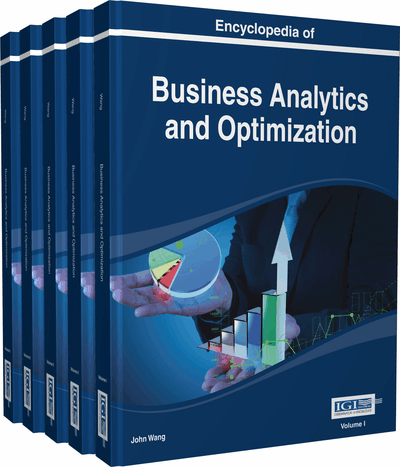# The Cosine Similarity in Terms of the Euclidean Distance

Marzena Kryszkiewicz (Warsaw University of Technology, Poland)
DOI: 10.4018/978-1-4666-5202-6.ch223

Top

## Introduction

In many applications, especially in information retrieval, text mining, biomedical engineering and chemistry, the cosine similarity is often used to find objects most similar to a given one, so called nearest neighbors. Objects are typically represented as vectors. In particular, documents are often represented as term frequency vectors or its variants such as tf_idf vectors (Salton, Wong, & Yang 1975; Han, Kamber, & Pei 2011). The cosine similarity measure between vectors is interpreted as the cosine of the angle between them. According to this measure, two vectors are treated as similar if the angle between them is sufficiently small; that is, if its cosine is sufficiently close to 1.

The determination of nearest neighbors is challenging if analyzed vectors are high dimensional. In the case of distance metrics, one may apply the triangle inequality to quickly prune large numbers of objects that certainly are not nearest neighbors of a given vector (Uhlmann, 1991, Moore, 2000; Elkan, 2003; Kryszkiewicz & Lasek, 2010a; Kryszkiewicz & Lasek, 2010b; Kryszkiewicz & Lasek, 2010c; Patra, Hubballi, Biswas & Nandi, 2010; Kryszkiewicz & Lasek, 2011). Nevertheless, the cosine similarity is not a distance metric and, in particular, does not preserve the triangle inequality in general. In spite of this fact it was shown recently in (Kryszkiewicz, 2011; Kryszkiewicz, 2013a) that the problem of determining a cosine similarity neighborhood can be transformed to the problem of determining the Euclidean distance. This result allows applying the triangle inequality to make the determination of cosine similarity neighborhoods faster.

The objective of this chapter is to present the ways in which the relationship between the cosine similarity and the Euclidean distance can be used to determine cosine similar objects efficiently. We also outline further research directions related to this task.

## Key Terms in this Chapter

e-Neighborhood: In the case of applying the Euclidean distance, e -neighborhood of a vector u is the set of all vectors that lie within e distance from vector u . In the case of applying the cosine similarity measure, e -neighborhood of a vector u is the set of all vectors such that their cosine similarity to vector u equals at least e .

The Cosine Similarity of Vectors: The cosine of the angle between vectors.

Normalized Form of a Vector: A normalized form of a vector u is the vector obtainable from vector u by dividing each component of vector u by the length of u .

The Euclidean Distance between Vectors: The Euclidean distance between two vectors equals the square root of the sum of the squared differences calculated between each pair of corresponding components of these vectors.

Weighted Binary Vector: Vector whose each dimension has a domain consisting of at most two values: zero and a non-zero real value.

ZPN-Vector: Vector whose each dimension has a domain consisting of at most three values: zero, a positive number and a negative number.

The Triangle Inequality: The property that holds for a function d if d ( u , r ) = d ( u , v ) + d ( v , r ) (or equivalently, d ( u , v ) = d ( u , r ) - d ( v , r )) for any arguments u , v , r of this function.

Length of a Vector: The square root of the sum of the squared components of a given vector. In other words, the Euclidean distance between a given vector and zero vector.

k Nearest Neighbors: In the case of applying the Euclidean distance, k nearest neighbors of a vector u in a set of vectors D are any k vectors v in D \ { u } such that the number of vectors in D \ { u } that are less distant from u than v does not exceed k - 1. In the case of applying the cosine similarity measure, k nearest neighbors of a vector u in a set of vectors D are any k vectors v in D \ { u } such that the number of vectors in D \ { u } the cosine similarity of which to vector u is greater than that of v does not exceed k - 1.

## Complete Chapter List

Search this Book:
Reset